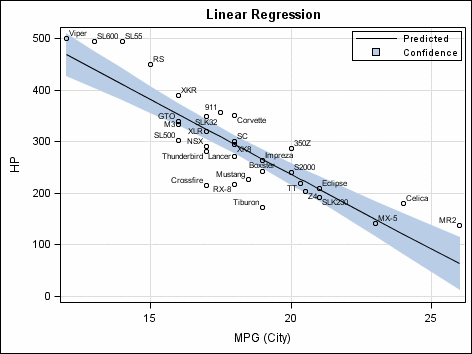# What is a linear regression?

Jun 3, 2014

In statistics, linear regression is an approach for modeling the relationship between a scalar dependent variable Y and one or more explanatory variables denoted X.

The case of one explanatory variable is called simple linear regression. For more than one explanatory variable, the process is called multiple linear regression.

In linear regression, data are modeled using linear predictor functions, and unknown model parameters are estimated from the data. Such models are called linear models.

This link contains an example of a linear regression question:

http://stattrek.com/regression/regression-example.aspx

Here's a graph of linear regression so you have an idea of what it looks like: